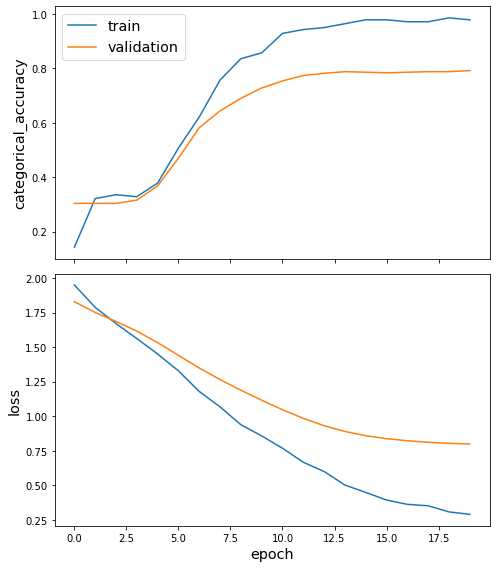# Interpreting nodes and edges with saliency maps in GCN¶

This demo shows how to use integrated gradients in graph convolutional networks to obtain accurate importance estimations for both the nodes and edges. The notebook consists of three parts: - setting up the node classification problem for Cora citation network - training and evaluating a GCN model for node classification - calculating node and edge importances for model’s predictions of query (“target”) nodes

References

 Axiomatic Attribution for Deep Networks. M. Sundararajan, A. Taly, and Q. Yan. Proceedings of the 34th International Conference on Machine Learning, Sydney, Australia, PMLR 70, 2017 (link).

 Adversarial Examples on Graph Data: Deep Insights into Attack and Defense. H. Wu, C. Wang, Y. Tyshetskiy, A. Docherty, K. Lu, and L. Zhu. arXiv: 1903.01610 (link).

:

import networkx as nx
import pandas as pd
import numpy as np
from scipy import stats
import os
import time
import stellargraph as sg
from stellargraph.mapper import FullBatchNodeGenerator
from stellargraph.layer import GCN
from tensorflow import keras
from tensorflow.keras import layers, optimizers, losses, metrics, Model, regularizers
from sklearn import preprocessing, feature_extraction, model_selection
from copy import deepcopy
import matplotlib.pyplot as plt
from stellargraph import datasets
from IPython.display import display, HTML
%matplotlib inline


:

dataset = datasets.Cora()
display(HTML(dataset.description))

The Cora dataset consists of 2708 scientific publications classified into one of seven classes. The citation network consists of 5429 links. Each publication in the dataset is described by a 0/1-valued word vector indicating the absence/presence of the corresponding word from the dictionary. The dictionary consists of 1433 unique words.

### Splitting the data¶

For machine learning we want to take a subset of the nodes for training, and use the rest for validation and testing. We’ll use scikit-learn again to do this.

Here we’re taking 140 node labels for training, 500 for validation, and the rest for testing.

:

train_subjects, test_subjects = model_selection.train_test_split(
subjects, train_size=140, test_size=None, stratify=subjects
)
val_subjects, test_subjects = model_selection.train_test_split(
test_subjects, train_size=500, test_size=None, stratify=test_subjects
)


### Converting to numeric arrays¶

For our categorical target, we will use one-hot vectors that will be fed into a soft-max Keras layer during training. To do this conversion …

:

target_encoding = preprocessing.LabelBinarizer()

train_targets = target_encoding.fit_transform(train_subjects)
val_targets = target_encoding.transform(val_subjects)
test_targets = target_encoding.transform(test_subjects)

all_targets = target_encoding.transform(subjects)


### Creating the GCN model in Keras¶

To feed data from the graph to the Keras model we need a generator. Since GCN is a full-batch model, we use the FullBatchNodeGenerator class.

Note: For interpretability we require a dense matrix so we set sparse=False in the FullBatchNodeGenerator.

:

generator = FullBatchNodeGenerator(G, sparse=False)

Using GCN (local pooling) filters...


For training we map only the training nodes returned from our splitter and the target values.

:

train_gen = generator.flow(train_subjects.index, train_targets)


Now we can specify our machine learning model: tn this example we use two GCN layers with 16-dimensional hidden node features at each layer with ELU activation functions.

:

layer_sizes = [16, 16]
gcn = GCN(
layer_sizes=layer_sizes,
activations=["elu", "elu"],
generator=generator,
dropout=0.3,
kernel_regularizer=regularizers.l2(5e-4),
)

:

# Expose the input and output tensors of the GCN model for node prediction, via GCN.in_out_tensors() method:
x_inp, x_out = gcn.in_out_tensors()
# Snap the final estimator layer to x_out
x_out = layers.Dense(units=train_targets.shape, activation="softmax")(x_out)


### Training the model¶

Now let’s create the actual Keras model with the input tensors x_inp and output tensors being the predictions x_out from the final dense layer

:

model = keras.Model(inputs=x_inp, outputs=x_out)

model.compile(
loss=losses.categorical_crossentropy,
metrics=[metrics.categorical_accuracy],
)


Train the model, keeping track of its loss and accuracy on the training set, and its generalisation performance on the validation set (we need to create another generator over the validation data for this)

:

val_gen = generator.flow(val_subjects.index, val_targets)


Train the model

:

history = model.fit(
train_gen, shuffle=False, epochs=20, verbose=2, validation_data=val_gen
)

Epoch 1/20
1/1 - 0s - loss: 1.9493 - categorical_accuracy: 0.1429 - val_loss: 1.8287 - val_categorical_accuracy: 0.3040
Epoch 2/20
1/1 - 0s - loss: 1.7875 - categorical_accuracy: 0.3214 - val_loss: 1.7499 - val_categorical_accuracy: 0.3040
Epoch 3/20
1/1 - 0s - loss: 1.6701 - categorical_accuracy: 0.3357 - val_loss: 1.6854 - val_categorical_accuracy: 0.3040
Epoch 4/20
1/1 - 0s - loss: 1.5617 - categorical_accuracy: 0.3286 - val_loss: 1.6155 - val_categorical_accuracy: 0.3160
Epoch 5/20
1/1 - 0s - loss: 1.4499 - categorical_accuracy: 0.3786 - val_loss: 1.5322 - val_categorical_accuracy: 0.3680
Epoch 6/20
1/1 - 0s - loss: 1.3278 - categorical_accuracy: 0.5071 - val_loss: 1.4399 - val_categorical_accuracy: 0.4700
Epoch 7/20
1/1 - 0s - loss: 1.1788 - categorical_accuracy: 0.6214 - val_loss: 1.3484 - val_categorical_accuracy: 0.5820
Epoch 8/20
1/1 - 0s - loss: 1.0673 - categorical_accuracy: 0.7571 - val_loss: 1.2649 - val_categorical_accuracy: 0.6440
Epoch 9/20
1/1 - 0s - loss: 0.9381 - categorical_accuracy: 0.8357 - val_loss: 1.1877 - val_categorical_accuracy: 0.6900
Epoch 10/20
1/1 - 0s - loss: 0.8570 - categorical_accuracy: 0.8571 - val_loss: 1.1152 - val_categorical_accuracy: 0.7280
Epoch 11/20
1/1 - 0s - loss: 0.7681 - categorical_accuracy: 0.9286 - val_loss: 1.0464 - val_categorical_accuracy: 0.7540
Epoch 12/20
1/1 - 0s - loss: 0.6665 - categorical_accuracy: 0.9429 - val_loss: 0.9840 - val_categorical_accuracy: 0.7740
Epoch 13/20
1/1 - 0s - loss: 0.5994 - categorical_accuracy: 0.9500 - val_loss: 0.9309 - val_categorical_accuracy: 0.7820
Epoch 14/20
1/1 - 0s - loss: 0.5016 - categorical_accuracy: 0.9643 - val_loss: 0.8893 - val_categorical_accuracy: 0.7880
Epoch 15/20
1/1 - 0s - loss: 0.4481 - categorical_accuracy: 0.9786 - val_loss: 0.8585 - val_categorical_accuracy: 0.7860
Epoch 16/20
1/1 - 0s - loss: 0.3930 - categorical_accuracy: 0.9786 - val_loss: 0.8370 - val_categorical_accuracy: 0.7840
Epoch 17/20
1/1 - 0s - loss: 0.3617 - categorical_accuracy: 0.9714 - val_loss: 0.8221 - val_categorical_accuracy: 0.7860
Epoch 18/20
1/1 - 0s - loss: 0.3515 - categorical_accuracy: 0.9714 - val_loss: 0.8109 - val_categorical_accuracy: 0.7880
Epoch 19/20
1/1 - 0s - loss: 0.3070 - categorical_accuracy: 0.9857 - val_loss: 0.8035 - val_categorical_accuracy: 0.7880
Epoch 20/20
1/1 - 0s - loss: 0.2896 - categorical_accuracy: 0.9786 - val_loss: 0.7987 - val_categorical_accuracy: 0.7920

:

sg.utils.plot_history(history)Evaluate the trained model on the test set

:

test_gen = generator.flow(test_subjects.index, test_targets)
test_metrics = model.evaluate(test_gen)
print("\nTest Set Metrics:")
for name, val in zip(model.metrics_names, test_metrics):
print("\t{}: {:0.4f}".format(name, val))


Test Set Metrics:
loss: 0.7585
categorical_accuracy: 0.8037